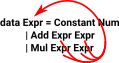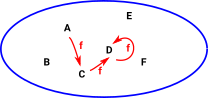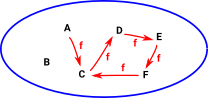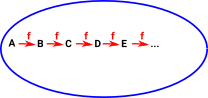# Maths - Recursive Types

#### Quotes

 Algebraic data type is least fixed point of a functor.Least fixed points are closely related to free algebras (site here).### Inductively and Co-inductively Defined Types

Inductive types coinductive types
Least Fixpoint or initial solution of isomorphism equations. Greatest Fixpoint or final solution of isomorphism equations.
Elements may be obtained by a finite composition of its introductory forms. Elements behave properly in response to a finite composition of elimination forms.
Recursor Generator
Catamorphism Anamorphism

An F-algebra is a triple consisting of:

• endofunctor F
• object a (carrier or underlying object)
• morphism F a -> a (evaluation function or the structure map)

where F forms expressions and the morphism as evaluates them.

 Idris definition of an F-algebra f is now lower case due to programming conventions. `interface Algebra f a = f a -> a`

### Algebra for a Functor

An algebra for a functor F is a pair <X, α>

where

• X is a type
• α : F X->X

### Example of Lists

 In most languages we can construct lists, for example here is a list of integers: ```Idris> [1,2] [1, 2] : List Integer``` We can also nest lists, here is a list of lists of integers: ```Idris> [[1,2],[3,4]] [[1, 2], [3, 4]] : List (List Integer)``` Sometimes we may need to have different depths in different parts of the list. This can be a problem as in this case: ```Idris> [[1,2],3] List Integer is not a numeric type```

What we need is a type where adding an inner type does not change the overall type, like this:

`MyList ( MyList x) = MyList x `

Where = means 'is the same type as'

This can easily be done with recursive types. We can analyse this by using the concept of a 'fixpoint'.

### Fixpoints

 endofunction example `int -> int ` Functions from a type to an element of the same type (endofunctions) can have fixpoints. More information about fixpoints on this page. endofunctor example ` Expr ->Expr` We can also have fixpoints between more complicated types. Inductivly Defined Type```data Expr = Constant Nat | Var String | Add Expr Expr | Mul Expr Expr``` Inductivly defined types can also have fixpoints.We have an endofunctor Expr which takes a type and gives us an expression over that type.

We can have an expression over some base:

Expr Base

and we can have an expression over that and so on:

• Base
• Expr Base
• Expr Expr Base
• Expr Expr ExprBase
• ...

The fixpoint is where Expr x = x

### Example of Fixpoint on Sets

In order to get some insight I think it helps to look at sets graphically:

 These examples show the action of an endofunction 'f' on some set. For example 'f' sends 'A' to 'C', 'C' to 'D' and 'D' to itself. So 'D' is a fixpoint.Some endofunctions may not have a fixpoint, in this example there is a loop around C,D,E and F.Or, if the set is infinite, there may not be any loops.So we can see from this that not every endofunction would have a fixpoint or there could be many fixpoints.

### Examples

Functor Initial Algebra Co-algebra
List X -> 1 + A × X List A = 1 + A × X
Natural Numbers X -> 1 + X N = 1 + X
Binary Tree X -> A + X × X
Stream X -> A × X

### Fixpoint Combinator using Idris

Fixed-point combinator Wiki

It would be good to have a function which takes an endofunction and generates a fixpoint from it (If it exists).

 So, this would be the signature of such a function: `ffix : (a -> a) -> a` Here is an implementation: It looks like this function would try to construct an infinite sequence of function calls f (f (f (f (f ...)))) and we would expect the program to run out of memory and crash. ```ffix : (a -> a) -> a ffix f {a} = x where x : a x = f x``` However, if the language can support lazy (as opposed to eager) types then, when the parameter is not used, the function will terminate. ```*fixPoint> ffix (const 3) 3 : Integer``` In this example we give it 'Id' which we would expect to cause an infinite loop. With this Idris example, it sometimes seems to detect this and to go into lazy mode: ```*fixPoint> ffix Id Fix.ffix, x Type Id : Type``` and this is also lazy. If returns a function instead of trying to evaluate an infinite loop. ```*fixPoint> ffix (+2) prim__addBigInt (Fix.ffix, x Integer ( \ARG => prim__addBigInt ARG 2)) 2 : Integer ```

Ideally we would like this function to return some special value (such as bottom) if there is no fixpoint. This would be more practical for types.

### Existence of Fixpoints - Monotonicity

A fixpoint is guaranteed to exist if the function is monotone (Knaster-Tarski fixpoint theorem).

That is, if there is an order on the elements and the function preserves the order.

This applies even if the order is a partial order provided there is a bottom element.

This order may be thought of in different ways depending if we are coming from the direction of denotional semantics or category theory:

Denotional Semantics Category Theory
Order

Applies to a partially defined function.

Lower means less well defined and higher means more defined.

Lower means toward 'initial' and higher means toward 'final'.
Bottom Element Denoted. This means undefined/error/non-terminating computation. The least fixed point is the fixed point with a morphism to every other morphism.

The semantics should not just be monotone but also continuous , in which the least fixpoint is the union of all finite iterates.

Functions for which f() =are called strict.

### Strictly Positive Functor

An inductive type is defined by a functor but not all functors can be used to define an inductive type.

A functor is strictly positive if it is defined by an expression that only uses constant types, the variable type X and the operators ×, +, ->, so that X only occurs to the right of arrows. Arrows (functions) are covarient in codomain and contravarient in domain.

### Fixpoint in Types

What we are more interested in here is fixpoints for functions between types (Type -> Type) that is a type that depends on another type such as 'List'.

 The intuition behind this definition is that, since we applied f infinitely many times to get Fix f, applying it one more time doesn’t change anything. `Fix f = f (Fix f)` In Haskell library it is defined like this: Both type name and constuctor are called Fix In Idris we need to make it lazy. ```||| Fix only has one constructor ||| (newtype in Haskell) ||| this is a recursive definition data Fix f = Fix (f (Fix f))```

#### Algebraic Data Type and Least Fixed Point of a Functor

 Algebraic data type least fixed point of a functor `data Fix f = Fix (f (Fix f))` ```ffix : (a -> a) -> a ffix f {a} = x where x : a x = f x```

#### Discussion on Idris Github

 Idris code example from here. ```module Main fix : (|(x: a) -> a) -> a fix f = x where x = f (lazy x) foo : |(x: Int -> Int) -> Int -> Int foo _ 0 = 0 foo f n = f (n - 1) main : IO main = print ((fix foo) 12)```

 another example from here Strictly Positive Functors When using Idris how can we ensure that the base functor of the datatype is strictly positive? Strictly positive functors can be represent by defining a universe or by containers. ```-- A universe of positive functor data Desc : Type where ||| sigma type Sig : (a : Type) -> (a -> Desc) -> Desc ||| a (strictly-positive) position for a recursive substructure Rec : Desc -> Desc ||| nothing End : Desc -- The decoding as a Type -> Type function desc : Desc -> Type -> Type desc (Sig a d) x = (v : a ** desc (d v) x) desc (Rec d) x = (x, desc d x) desc End x = ()``` From this universe of functors, which are guaranteed to be strictly positive, we can take their least fixpoint: ```-- The least fixpoint of such a positive functor data Mu : Desc -> Type where In : desc d (Mu d) -> Mu d``` Example: Nat Start with a sum type. `data NatC = ZERO | SUCC` We then define the strictly positive base functor by storing the constructor tag in a sigma and computing the rest of the description based on the tag value. The ZERO tag is associated to End (there is nothing else to store in a zero constructor) whilst the SUCC one requires a Rec End, that is to say one recursive substructure corresponding to the Nat's predecessor. ```natD : Desc natD = Sig NatC \$ \ c => case c of ZERO => End SUCC => Rec End``` Inductive type by taking the fixpoint of the description: ```nat : Type nat = Mu natD``` Recover the constructors: ```zero : nat zero = In (ZERO ** ()) succ : nat -> nat succ n = In (SUCC ** (n, ()))``` References This specific universe is taken from 'Ornamental Algebras, Algebraic Ornaments' by McBride but you can find more details in 'The Gentle Art of Levitation' by Chapman, Dagand, McBride, and Morris.

### Computer Implementation of Algebra

Requires a carrier type to be defined then we need the algebra to be defined like this:

 Monoid `data MonF a = MEmpty | MAppend a a ` Ring `data RingF a = RZero | ROne | RNeg a | RAdd a a | Rmull a a `

To make this into an expression we need a recursive definition :

 Monoid `data MonExpr = MEmpty | MAppend Expr Expr ` Ring ```data RingExpr = RZero | ROne | RNeg RingExpr | RAdd RingExpr RingExpr | Rmull RingExpr RingExpr ```

Other sites

Where I can, I have put links to Amazon for books that are relevant to the subject, click on the appropriate country flag to get more details of the book or to buy it from them.Computation and Reasoning - This book is about type theory. Although it is very technical it is aimed at computer scientists, so it has more discussion than a book aimed at pure mathematicians. It is especially useful for the coverage of dependant types.